# Solve Kakuro puzzles

In this guide we offer a range of practical tips on how to solve Kakuro puzzles, with difficulties ranging from beginner to expert level.

To summarize the rules: Kakuro is a puzzle game on a crosswords-like board where digits are used in order to make them sum up to values specified in the 'definition' squares of the board. In addition, inside each sum group, each digit can appear once at most.

The traditional way to solve a Kakuro puzzle is incremental: by using the existing information on the board, you can find with certainty the value of a specific cell which can take only one possible value. Then that value is filled and the process is repeated until all the board cells have been discovered.

In some situations, there's no specific board cell having just one possibility. In those cases each of the possibilities needs to be explored on its own and eliminated through contradictions until only one course of action remains.

We're presenting below several methods to make progress in solving the actual puzzle.

## Unique sums for specific definitions

There are some definitions that can be solved only in a specific way:

• the sum 3 (across two cells) will always be 1 + 2.
• the sum 4 (across two cells) will always be 1 + 3.
• the sum 17 (across two cells) will always be 8 + 9.
• the sum 6 (across three cells) will always be 1 + 2 + 3.
• the sum 24 (across three cells) will always be 7 + 8 + 9.

and so on... Usually you can hover on a Kakuro grid over the definition number and a tooltip will appear containing all the possibilities of writing that sum with unique digits in the number of available cells.

The sums that can be written in an unique way are usually low-sums or high-sums which force low/high digits in the answer in order to attain them.

Having an unique way of writing the sum helps, but keep in mind that all permutations are valid and you still need to figure out which actual permutation to use on the board.

## Row / column restrictions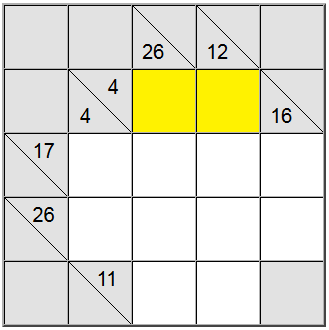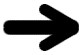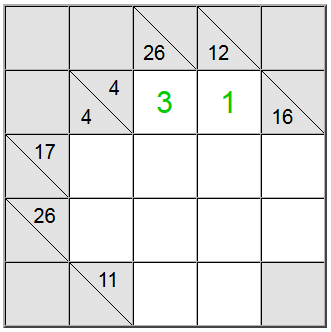For the yellow cells highlighted above, there's only one way of writing the sum: 4 = 1 + 3. However we still need to figure out which permutation (1 + 3 or 3 + 1) to use.

Turns out that the 26 vertical definition helps us: a sum across 4 cells that would contain the digit 1 would be at most 1 + 9 + 8 + 7 = 25. Since our sum is 26, it turns out that the digit 1 cannot be a part of the sum. Therefore, the only remaining order for the yellow squares is 3 + 1.

## Definitions intersections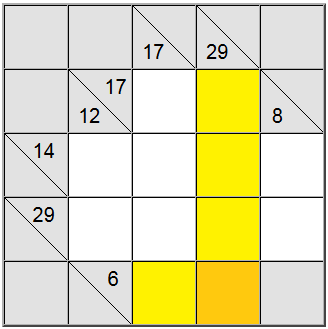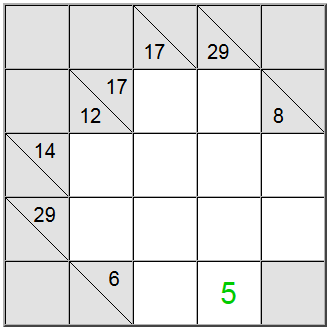On the board above, the horizontal yellow squares can be written as 6 = 1 + 5 or 6 = 2 + 4. The vertical yellow sum can only be written as 29 = 5 + 7 + 8 + 9.

The yellow square found at the intersection of those two sum definitions must contain the same digit, so there must be a common digit present in the horizontal and vertical definitions in order to be shared between them. By looking at the possibilities above we can easily figure out that 5 is the only digit which respects this criterion.

This technique works especially well when intersecting a low-sum with a high-sum definition. Low-sum and high-sum definitions are the ones which have a relative low or high sum definition number (6 and 29 respectively for our example) when compared to the number of cells available. Because 6 is relatively low it will force low digits in the sum representation, and 29 will force high digits (in order to attain these sums using the given number of cells). Therefore the intersection of low and high digits is likely to contain only one candidate for the actual cell value.

## Min / max values for a sum group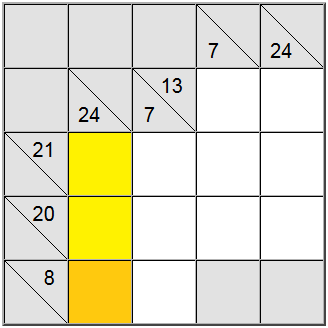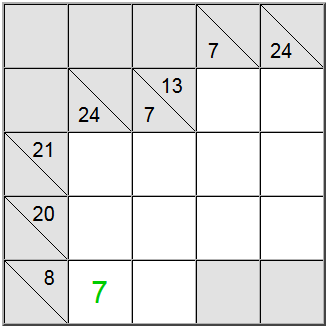Sometimes it's useful to figure out the max or min value for a sum group. This can give you a sense of what's the valid range of digits for that particular sum, which in turn will help you make correlation with other restrictions and figure out the unique assignments on the board.

In the example above, the yellow vertical cells only accept values equal to 7 or higher. If you try to assign a 6, you can easily figure out that 6 + 9 + 8 = 23 so the definition sum of 24 would not be attainable.

Because 7 is a minimum, the horizontal definition having 8 as a sum forces the 7 in that position.

## Conclusion

Practicing is the best way to see how this advice can be applied in actual Kakuro games. Play a puzzle for real. We wish you good luck and lots of fun!

© 2023 - All rights reserved - Contact Page - Privacy Policy - DE | EN | ES | FR | IT | JA | LV | NL | PL | RO | SV | TR